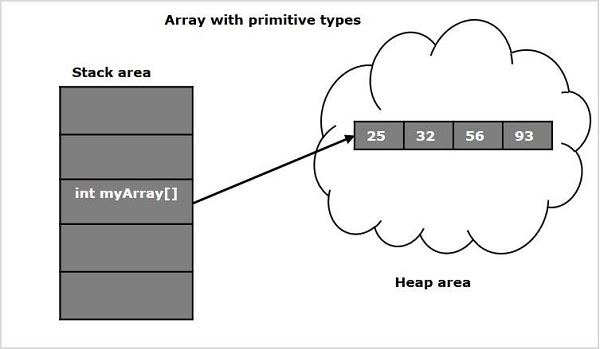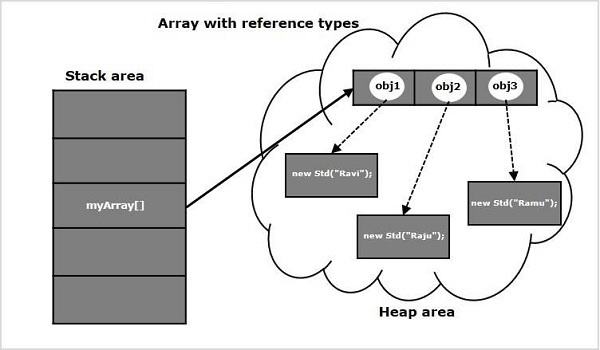# Where does Array stored in JVM memory in Java?

Array is a container which can hold a fix number of entities, which are of the of the same type. Each entity of an array is known as element and, the position of each element is indicated by an integer (starting from 0) value known as index.

## Example

import java.util.Arrays;
public class ArrayExample {
public static void main(String args[]) {
Number integerArray[] = new Integer;
integerArray = 25;
integerArray = 32;
integerArray = 56;
System.out.println(Arrays.toString(integerArray));
}
}

## Output

[25, 32, 56]

Arrays are two types −

• Single dimensional arrays: Normal arrays.
• Multi-dimensional arrays: Arrays storing arrays.

## JVM memory locations

JVM has five memory locations namely −

• Heap − Runtime storage allocation for objects (reference types).
• Stack − Storage for local variables and partial results. A stack contains frames and allocates one for each thread. Once a thread gets completed, this frame also gets destroyed. It also plays roles in method invocation and returns.
• PC Registers − Program Counter Registers contains the address of an instruction that JVM is currently executing.
• Execution Engine − It has a virtual processor, interpreter to interpret bytecode instructions one by one and a JIT, just in time compiler.
• Native method stacks − It contains all the native methods used by the application.

## Storage of Arrays

As discussed, the reference types in Java are stored in heap area. Since arrays are reference types (we can create them using the new keyword) these are also stored in heap area.In addition to primitive datatypes arrays also store reference types: Another arrays (multi-dimensional), Objects. In this case the object array/multi-dimensional stores the references of the objects/arrays init, which points to the location of the objects/arrays.

For suppose, if we have a class with name Std with constructor that accepts the name of a student and if we have defined an array of this class and populated it as shown below.

class Std {
private String name;
public Std(String name){
this.name = name;
}
}
public class Sample {
public static void main(String args[]) throws Exception {
//Creating an array to store objects of type Std
Std myArray[] = new Std;
//Populating the array
myArray  = new Std("Ravi");
myArray  = new Std("Raju");
myArray  = new Std("Ramu");
}
}

The memory of the array myArray might be like −Updated on: 02-Jul-2020

3K+ Views